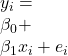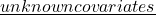# Quasi-empirical Bayes modeling of measurement error models and R-estimation of the regression parameters

This paper deals with the R-estimation of the regression parameters of a mea-
surement error model:and,By combining the two sets of the information, an emaculate regression model is obtained
using “quasi-empirical Bayes” estimates of thex_1, \ldots, x_n\$.
The model produces consistent estimates of the attenuated slope and the inter-
cept parameters and applies to broad range of regression problems. Asymptotic
properties of the R-estimators are provided based on the \quasi-Bayes regression
model”. Some simulated results are presented as evidence of the performances of
the estimators.

44n2_7